# Impulse of Force

The product of average force and the time it is exerted is called the impulse of force. From Newton's second law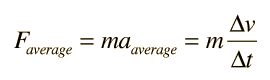the impulse of force can be extracted and found to be equal to the change in momentum of an object provided the mass is constant: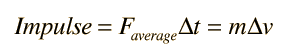Calculation
The main utility of the concept is in the study of the average impact force during collisions. For collisions, the mass and change in velocity are often readily measured, but the force during the collision is not. If the time of collision can be measured, then the average force of impact can be calculated.

 Minimizing impact force Airplane and duck collision Big truck, small truck collision
 Application in Rutherford scattering experiment
Index

Collision concepts

 HyperPhysics***** Mechanics ***** Newton's laws R Nave
Go Back

# Minimizing Impact Force

The process of minimizing an impact force can be approached from the definition of the impulse of force: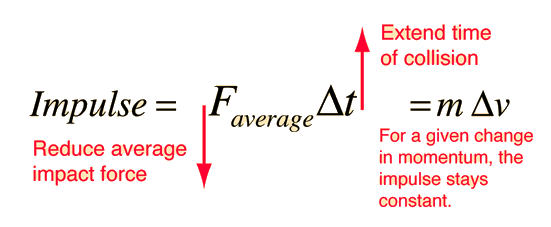If an impact stops a moving object, then the change in momentum is a fixed quantity, and extending the time of the collision will decrease the time average of the impact force by the same factor. This principle is applied in many common-sense situations:

• If you jump to the ground from any height, you bend your knees upon impact, extending the time of collision and lessening the impact force.
• A boxer moves away from a punch, extending the time of impact and lessening the force.
• Automobiles are made to collapse upon impact, extending the time of collision and lessening the impact force.

Alternatively, the same scenario can be examined with the aid of the work-energy principle.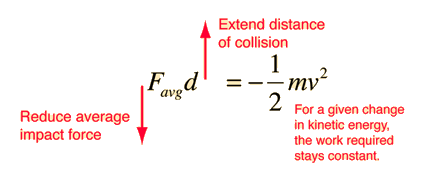Car crash example

An impact which stops a moving object must do enough work to take away its kinetic energy, so extending the distance moved during the collision reduces the average impact force.

Note: this approach to minimizing force during a collision uses the average of force over distance rather than the average over time. The profile of force during a collision such as that of a car with a tree can be complex, whether expressed in terms of time or distance.

 How do you define average force?
Index

Collision concepts

 HyperPhysics***** Mechanics ***** Newton's laws R Nave
Go Back

# What do you mean by average force?

The net external force on a constant mass object obeys Newton's second law, Fnet external=ma. The most straightforward way to approach the concept of average force is to multiply the constant mass times the average acceleration, and in that approach the average force is an average over time. Another approach that is valuable for assessing the impact force in high speed collisions is to use the impulse of force. When you strike a golf ball with a club, if you can measure the momentum of the golf ball and also measure the time of impact, you can divide the momentum change by the time to get the average force of impact. This average force is also an average over time.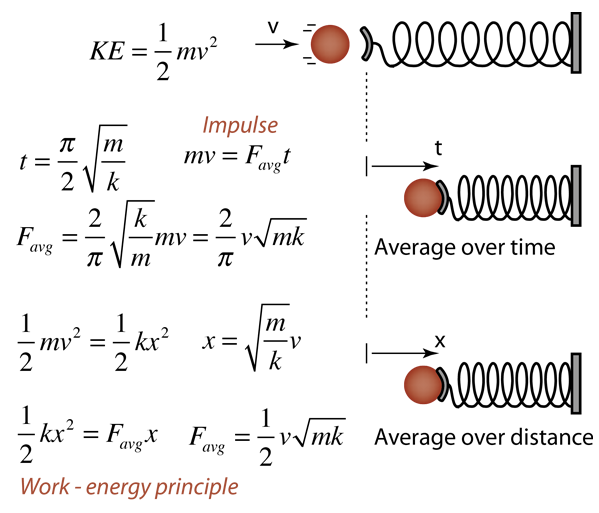There are, however, situations in which the distance traveled in a collision is readily measured while the time of the collision is not. In a case like a car crash with a tree, one can measure the distance of the collision and use the work-energy principle to evaluate the average of the force over the distance. Such an average over distance is not the same as the time average of force, but it is nevertheless useful in a case like evaluating the wisdom of wearing your seatbelt at all times in your automobile because without it, your stopping distance in a collision will be much shorter, and the average force on you much greater.

In the example illustrated above, a ball is brought to rest by impact with a spring. This provides an opportunity to evaluate the force average over time and the force average over distance. To make the comparison, the nature of simple harmonic motion is used, and the time to bring the ball to rest is one-fourth of a period of the periodic motion. The elastic motion would follow Hooke's law with a force constant k. The work-energy principle may also be applied, using the fact that in stopping the ball, the kinetic energy would be converted to spring potential energy. It is clear that the two approaches to an average force are not the same, although in this physical system they are proportional.

To provide a numerical example, consider a 1 kg mass traveling at 10 m/s that strikes a spring with spring constant k=10 N/m. This system would have a natural oscillation period of 1.99 seconds. So the time to stop the mass would be about a half second and the time average of force would be 20.1 Newtons. Using the work-energy principle to evaluate the distance average gives 15.8 Newtons.

Index

Collision concepts

Physics Forums

 HyperPhysics***** Mechanics ***** Newton's laws R Nave
Go Back

# Airplane and Duck

Estimate the average impact force between an airliner traveling at 600 mi/hr and a 1 pound duck whose length is 1 foot. This is an example of the use of impulse of force.### How much impact force?

Index

Collision concepts

 HyperPhysics***** Mechanics ***** Newton's laws R Nave
Go Back

# Airplane & Duck Force Estimate

For the airplane and duck force estimate, the mass of the duck is needed, but the weight in the U. S. Common system of units is given. The mass isRationale for estimates How can you reduce impact force? Variation of parameters

Jack Elrod in his Mark Trail comic strip (2/27/2000) says "If an aircraft strikes a big bird at a speed of 500 MPH, the impact will be about 25 tons." He is pretty well on target, considering the above model for a headon collision with a medium sized bird. The comment is in a strip on bird collisions in Israel, which has the world's largest density of migrating birds during the migration season. Since 1972 they have logged 1,282 "bird hits" with fighter aircraft, 696 with helicopters, and 637 with transport planes and light aircraft.

Index

Collision concepts

 HyperPhysics***** Mechanics ***** Newton's laws R Nave
Go Back

# Airplane & Duck Estimates

For the airplane and duck force estimate, the mass of the duck is determined, but the change in velocity and time of collision must be estimated in order to estimate the average impact force.

The change in velocity of the duck is estimated to be 600 mi/hr = 880 ft/s by assuming a head on collision, assuming that the duck is riding with the airliner after the collision, and assuming that the duck's velocity is negligible compared to that of the airliner, the "hovering duck" approximation.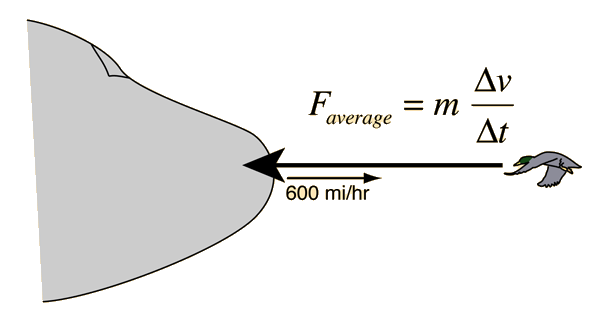The time of collision is assumed to be the time of transit of the duck's dimension of 1 foot, so 1/880 second.

Index

Collision concepts

 HyperPhysics***** Mechanics ***** Newton's laws R Nave
Go Back

# Airplane & Duck Collision Force

As a variation on the airplane and duck problem, change the mass of the bird and speed of the aircraft. Enter a length of the bird for estimation of the impact time.

Mass of bird m= kg, weight = N = lb.
Velocity of aircraft = m/s = km/h = mi/h, which for bird length = cm gives an estimated impact time of seconds. Under these conditions, the impact force is
Average impact force = N = lb = tons.Examine the case for a glancing collision
Index

Collision concepts

 HyperPhysics***** Mechanics ***** Newton's laws R Nave
Go Back